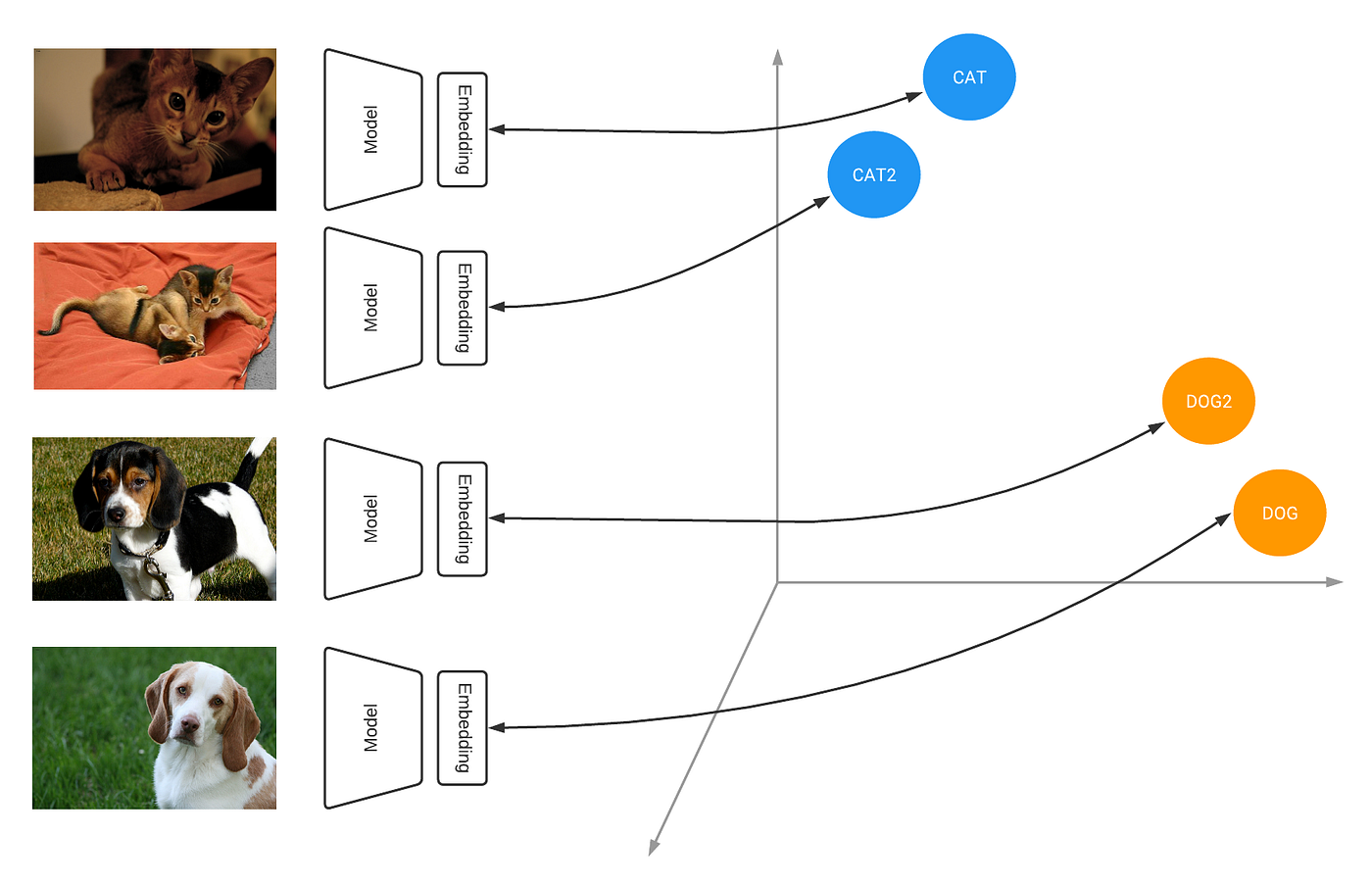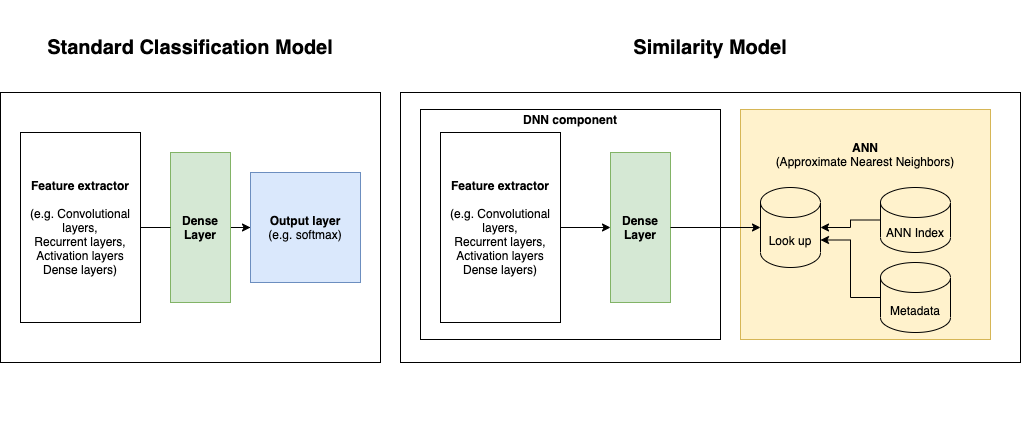# Tensorflow Similarity Model (Part 1) — what is it and how is it different from a normal classifier?Illustration of the metric space learned by Similarity Models (source: Introducing Similarity Model)

# Where can Similarity Model be useful?

Similarity Model provides the ability to search for related items. Its use cases are similar to the applicability of K-Nearest Neighbors search, but with the advantage of using DNN model as the input for the search domain, instead of raw data.

# Normal Deep Neural Network (DNN) Classifier vs Similarity Model

One might ask what is the difference between Similarity Model and normal DNN classification models. In short, Similarity Model can also be seen as a normal classification model (e.g. convolutional layers + activation layers + dense layers, etc.), but with different training objectives (different loss functions) and a different output handling.Diagram 1. Standard Classification Model vs Similarity Model.

## Training objectives in Similarity Model

Similarity Model focuses on contrastive learning, which learns output embeddings such that similar samples stay close together, and far from dissimilar ones. Therefore, unlike loss functions in standard classification models, which focus on the predictability of the classes in a dataset, Similarity Model uses a different set of loss functions called contrastive losses (e.g. Ranking loss, Marging loss, Hinge loss, etc.). The main goal of contrastive losses are to minimize the distance between similar samples, and maximize the distance between dissimilar samples.

## Model output

Output layer for normal classification models is usually a fully connected layer which can then be normalized using softmax or sigmoid function. The final output can be used directly for making predictions.

# Performing Nearest Neighbor search on the extracted features from a normal classifier?

Since the architecture for feature extraction can be shared between normal classifier and Similarity Models, one might ask if it is possible to perform nearest neighbors search on the model feature extraction output instead of training the metric embeddings a Similarity Model?

## Accuracy and speed

The distance between embedded points in Similarity Models use valid distance functions, which make it possible to perform Approximate NN. However, it is untrue in normal classifiers.

## Adding new classes

Adding new classes in Similarity Model can simply done by feeding new classes’ data into the trained model, adding the metric embeddings to the look-up and update the ANN index, metadata (in diagram 1). However, in normal classifiers, it might require model retraining since the model is fine-tuned towards the current classes.

--

--